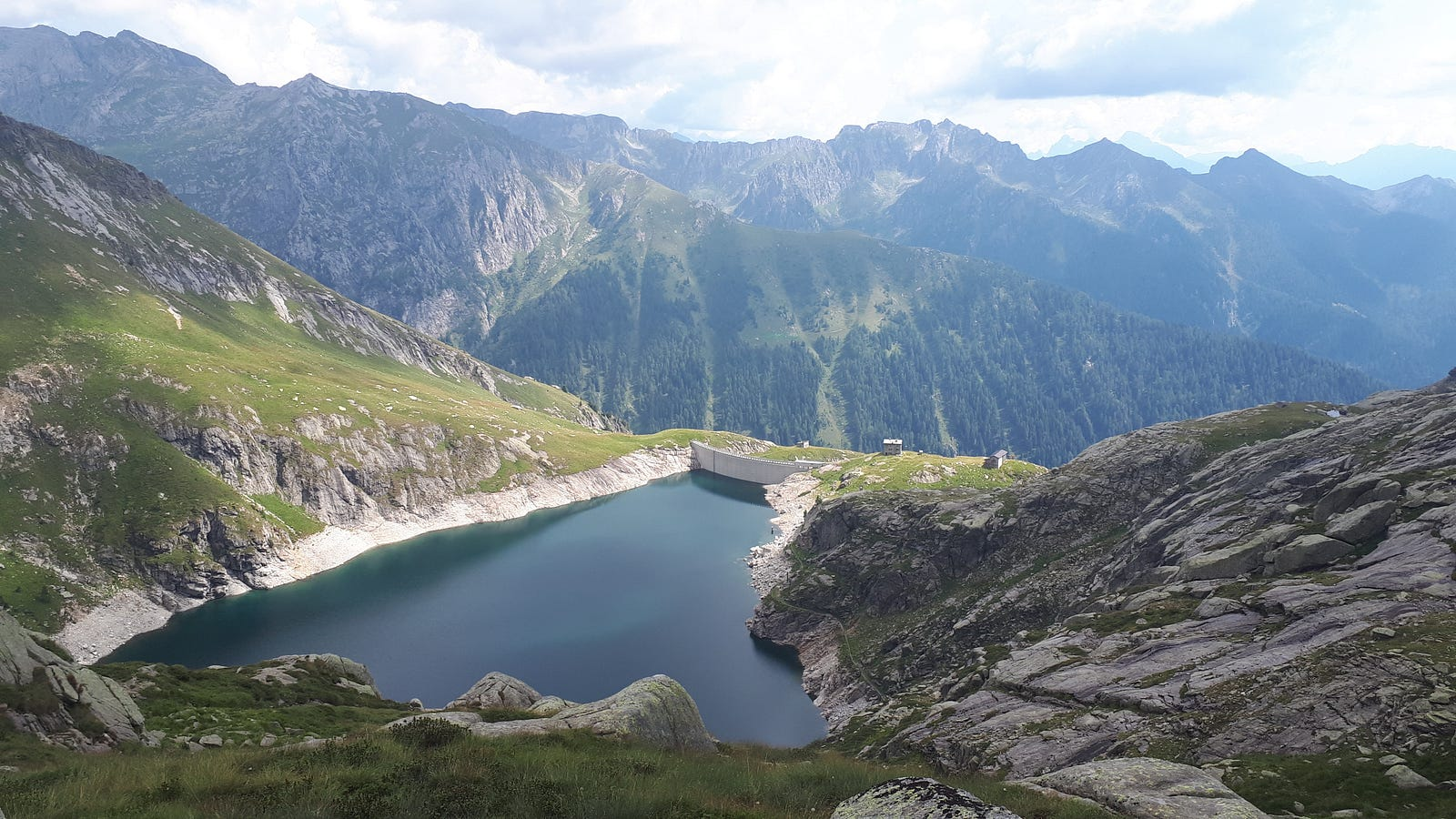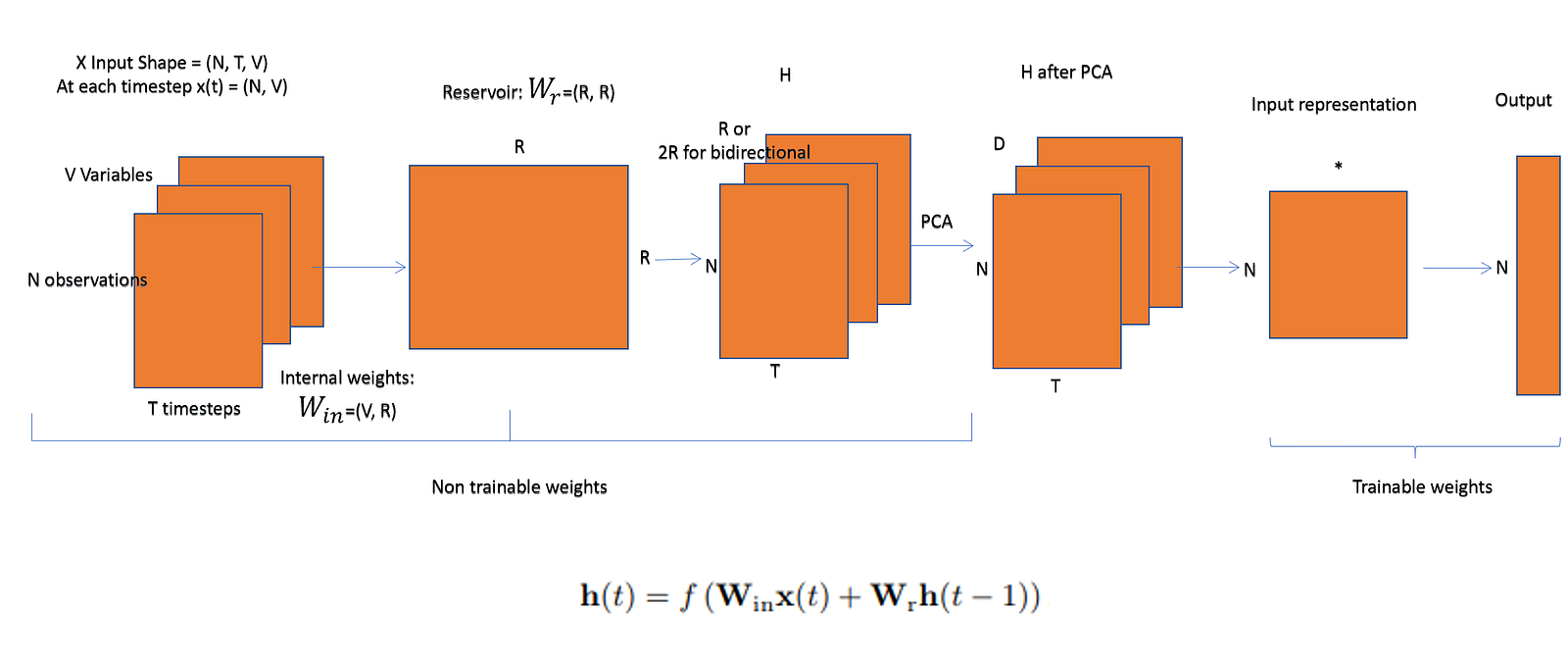Search

# Gentle introduction to Echo State NetworksThis post will address the following questions:

- What are Echo State Networks?

- Why and when should you use an Echo State Network?

- Simple implementation example in python

The figure below is a simplification of the paper Reservoir computing approaches for representation and classification of multivariate time series but it captures well the gist of ESNs. Each component will be detailed in the following sections.Echo State Networks are recurrent networks. f is a nonlinear function (such as tanh) which makes the current state dependent on the previous state and the current input

Echo state network is a type of Recurrent Neural Network, part of the reservoir computing framework, which has the following particularities:

- the weights between the input -the hidden layer ( the ‘reservoir’) : Win and also the weights of the ‘reservoir’: Wr are randomly assigned and not trainable

- the weights of the output neurons (the ‘readout’ layer) are trainable and can be learned so that the network can reproduce specific temporal patterns

- the hidden layer (or the ‘reservoir’) is very sparsely connected (typically < 10% connectivity)

- the reservoir architecture creates a recurrent non linear embedding (H on the image below) of the input which can be then connected to the desired output and these final weights will be trainable

- it is possible to connect the embedding to a different predictive model (a trainable NN or a ridge regressor/SVM for classification problems)

## Reservoir Computing

Reservoir computing is an extension of neural networks in which the input signal is connected to a fixed (non-trainable) and random dynamical system (the reservoir), thus creating a higher dimension representation (embedding). This embedding is then connected to the desired output via trainable units.

The non-recurrent equivalent of reservoir computing is the Extreme Learning Machine and consists only of feed forward networks having only the readout layer trainable.

## Workflow

For an input of shape N, T, V, where N is the number of observations, T is the number of time steps and V is the number of variables we will:

- choose the size of the reservoir R and other parameters governing the level of sparsity of connection, if we want to model a leakage, the ideal number of components after the dimensionality reduction, etc

- generate (V, R) input weights Win by sampling from a random binomial distribution

- generate (R, R) reservoir weights Wr by sampling from an uniform distribution with a given density, parameter which sets the level of sparsity

- calculate the high dimensional state representation H as a non linear function (typically tanh) of the input at the current time step (N, V) multiplied by the internal weights plus the previous state multiplied by the reservoir matrix (R, R)

- optionally we can run a dimensionality reduction algorithm such as PCA to D components, which brings H to (N, T, D)

-create an input representation either by using for example the entire reservoir and training a regressor to map states t to t+1: one representation could be the matrix of all calculated slopes and intercepts. Another option could be to use the mean or the last value of H

-connect this embedding to the desired output, either by using a NN structure which will be trainable or by using other types of predictors. The above mentioned paper suggest the use of Ridge regression

## Why and when should you use Echo State Networks?

-Traditional NN architectures suffer from the vanishing/exploding gradient problem and as such, the parameters in the hidden layers either don’t change that much or they lead to numeric instability and chaotic behavior. Echo state networks don’t suffer from this problem

-Traditional NN architectures are computationally expensive, Echo State Networks are very fast as there is no back propagation phase on the reservoir

-Traditional NN can be disrupted by bifurcations

-ESN are well adapted for handling chaotic time series

## Implementation

The paper comes with a cleanly written and well documented implementation of a pipeline proposing various approaches for each step (weight initialization scheme, dimensionality reduction, input representation, readout layer). I have created more simplified version by cherry-picking steps such as PCA, reservoir representation based on ridge regression and linear regression connection to output as these options were best performing on my input. I made this version available here.

### References

https://github.com/FilippoMB/Reservoir-Computing-framework-for-multivariate-time-series-classification

https://www.researchgate.net/post/what_is_the_realitionship_between_deep_learning_methods_and_reservoir_computing_if_any

See All